# Iwahori-Hecke algebra of symmetric group:S3

The Iwahori-Hecke algebra of symmetric group:S3 over a commutative unital ring$R$ is defined as the$R[q]$-algebra:$R[q] = \langle T_1,T_2 \rangle/ \langle (T_1 - q)(T_1 + 1), (T_2 - q)(T_2 + 1), T_1T_2T_1 - T_2T_1T_2 \rangle$

Specializing to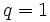$q = 1$ gives the group algebra over$R$ of symmetric group:S3:$R[S_3] = R\langle T_1,T_2,T_3 \rangle/\langle T_1^2 - 1, T_2^2 - 1, T_1T_2T_1 - T_2T_1T_2 \rangle$

The thing below needs to be fixed

Element$()$$\! T_1 \sim (1, 2)$$\! T_2 \sim (2, 3)$$\! T_1T_2T_1 \sim (1,3)$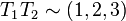$\! T_1T_2 \sim (1, 2, 3)$$\! T_2T_1 \sim (1, 3, 2)$$()$$()$$(1, 2)$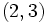$(2, 3)$$(1,3)$$(1, 2, 3)$$(1, 3, 2)$$\! T_1 \sim(1, 2)$$(1,2)$$q() + (q - 1)(1,2)$$q(1, 2, 3) + (q - 1)(1,3)$$q(1, 3, 2) + (q - 1)(1,2,3)$$q(2, 3) + (q - 1)(1,2,3)$$(1, 3)$$\! T_2 \sim (2, 3)$$(2,3)$$(1,3,2)$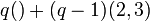$q() + (q - 1)(2,3)$$q(1, 2, 3) + (q - 1)(1,3)$$(1, 3)$$q(1, 2) + (q - 1)(1,3,2)$$\! T_1T_2T_1 \sim (1,3)$$(1,3)$$q(1, 2, 3) + (q - 1)(1,3)$$q(1, 3, 2) + (q - 1)(1,3)$  ?  ?  ?$\! T_1T_2 = (1, 2, 3)$$(1, 2, 3)$$(1,3)$$q(2, 3) + (q - 1)(1,2,3)$  ?  ?$\! T_2T_1 = (1, 3, 2)$$(1, 3, 2)$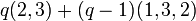$q(2, 3) + (q - 1)(1,3,2)$$(1, 3)$  ?  ?  ?